# Create calculated fields and measures

### Prerequisites

To assist with your learning, it is recommended that you look over the articles on the basics of Benevity Reporting in the following section:

### What are calculated fields and calculated measures?

In the ad hoc environment within Benevity Reporting, you can create custom fields and measures by applying formulas to existing fields and measures. This is helpful when you’re looking to segment your data or create calculated metrics based on your data. You can drag and drop the calculated fields and measures into tables, crosstabs, charts and filters and immediately view the results. Note: Some calculated fields and measures may not be available for use with filters.

• Fields: Dimensional or category data that you can apply to slice and segment the measures below.
• Calculated Fields: Dimensional or category data that has been modified by applying a formula or calculation.
• Measures: The numeric metrics used to measure and evaluate your program. These can be sliced and segmented by the various fields above.
• Calculated Measures: Numeric metrics that have been modified by applying a formula or calculation.

### Creating a calculated field

1. Create a new ad hoc view or open an existing ad hoc view that you would like to apply the calculated field to.

2. In the ad hoc editor, click on the three vertical dots to the right of Fields in the left-hand pane.

3. Click Create Calculated Field…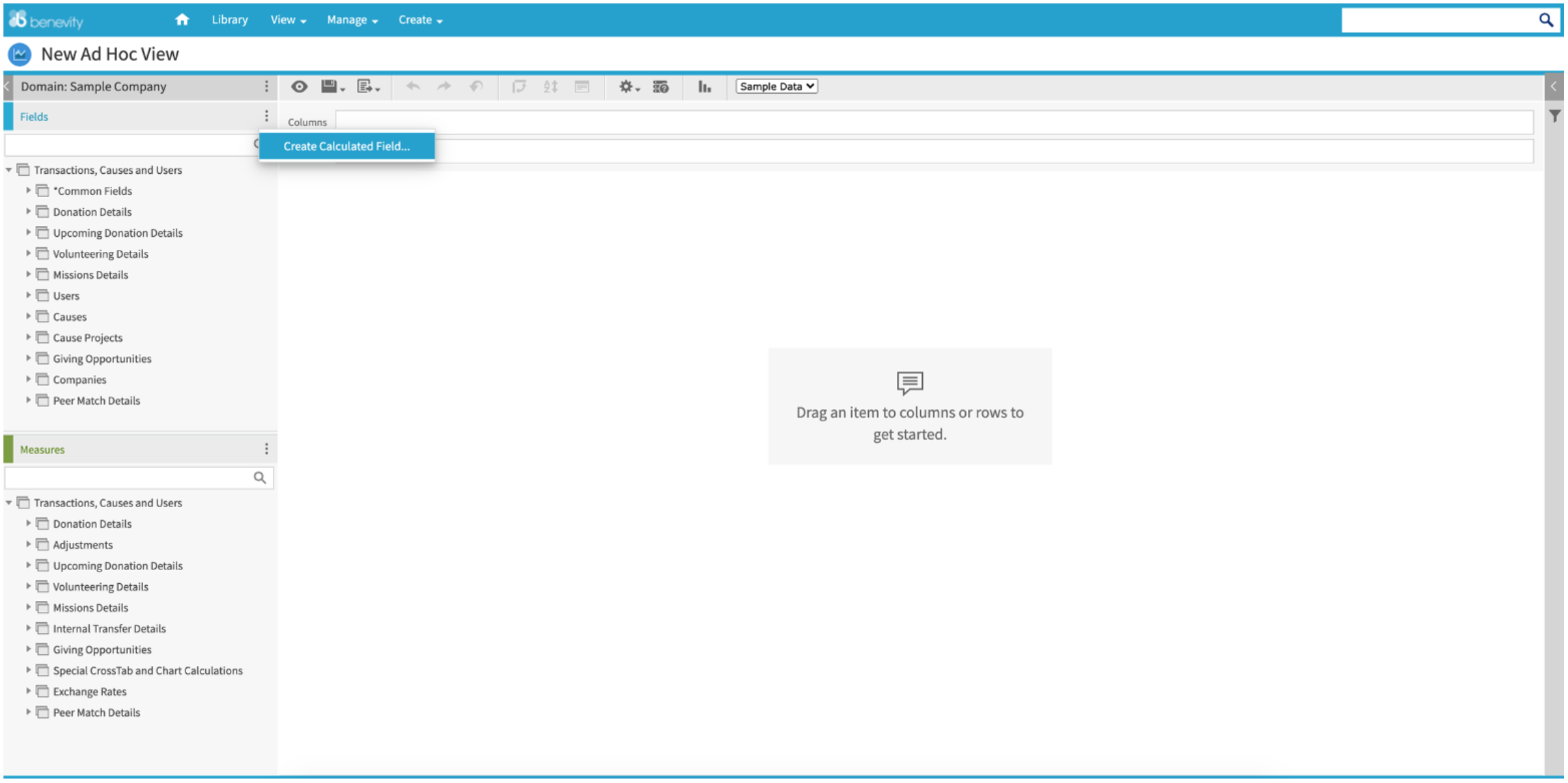In the middle of the screen, you will see the New Calculated Field window.

In the Formula Builder tab, you can create a custom formula by selecting a field from the list of Fields and Measures below and applying a formula to the field. A list of the various Functions available is also provided. You can see a short description of your chosen function in the Function Description view to the right of the Functions list.

As well, you can include various operators in the formula:

• Arithmetic operators (+ add, - subtract, * multiply, / divide)
• Boolean operators (AND, OR, NOT, IN)
• Other operators (== equals, != not equal, > greater than, < less than, >= greater than or equal to, <= less than or equal to)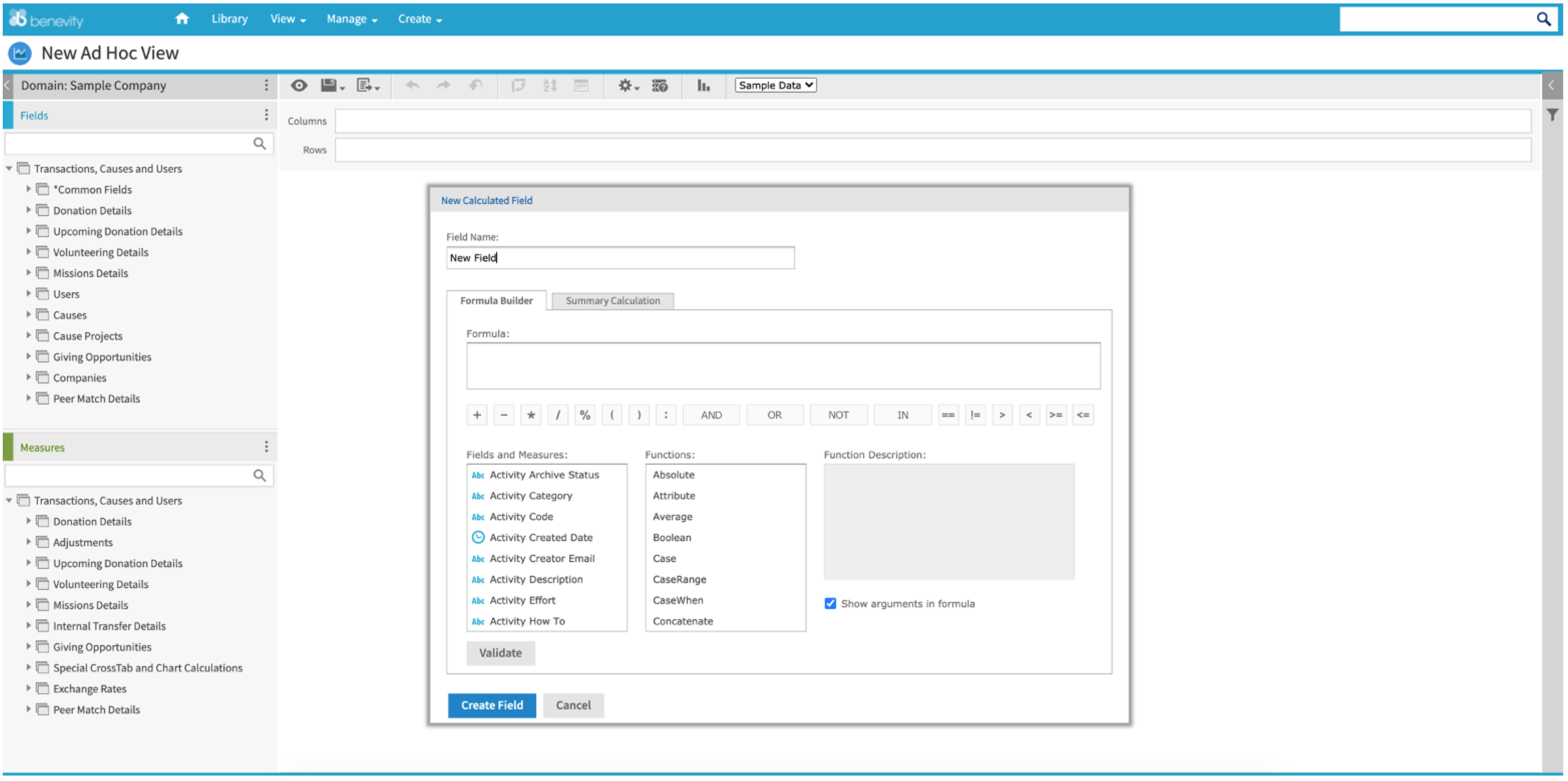5. Once you have created your calculated field, add a Field Name and click Validate to check that the formula has been created correctly.

6. Click Create Field.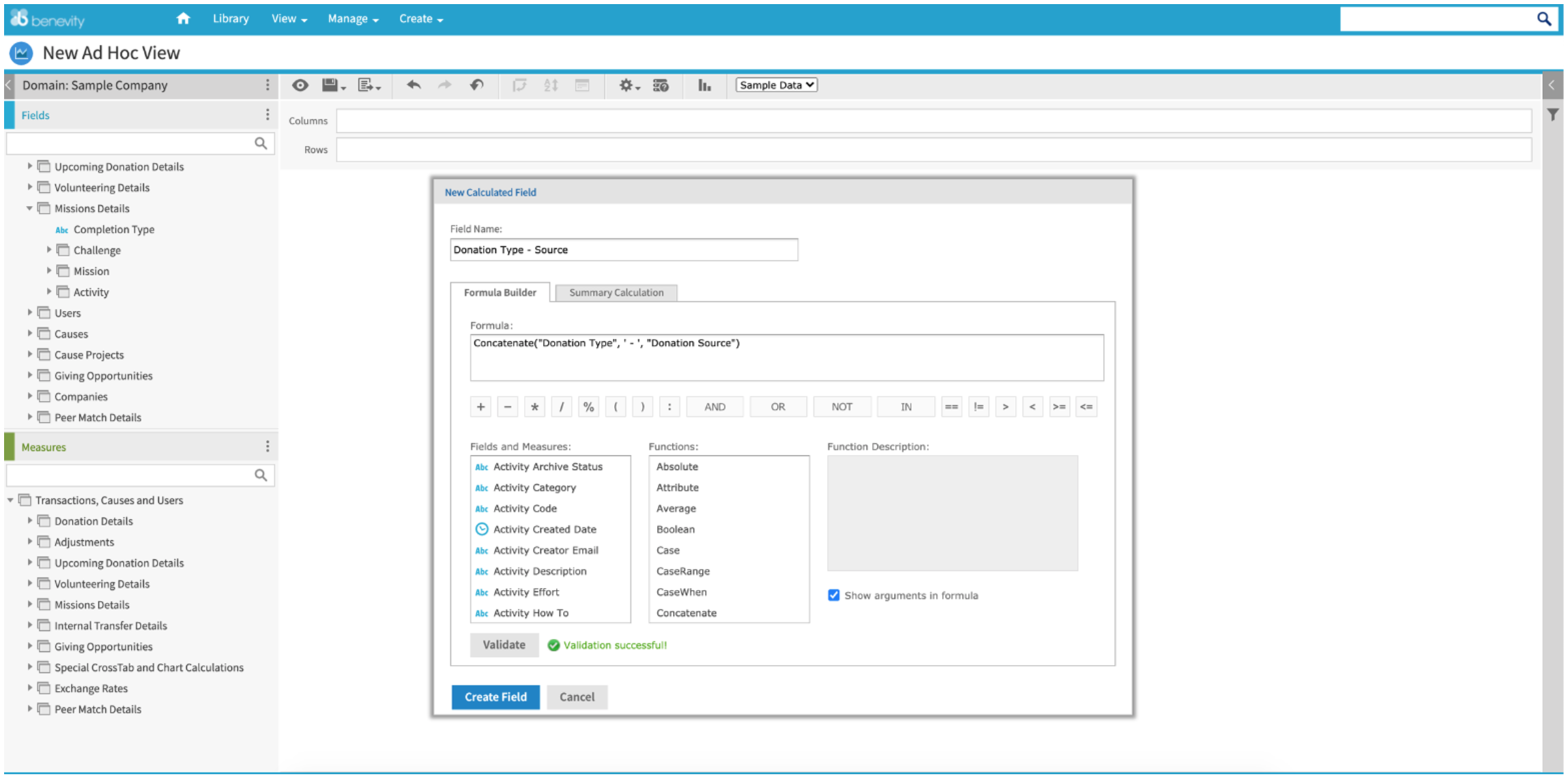Your new calculated field will appear at the bottom of your list of Fields and can now be dragged into the Columns or Rows section of the crosstab view.

7. Add the new calculated field to the Columns or Rows in the crosstab to view the output of your new field.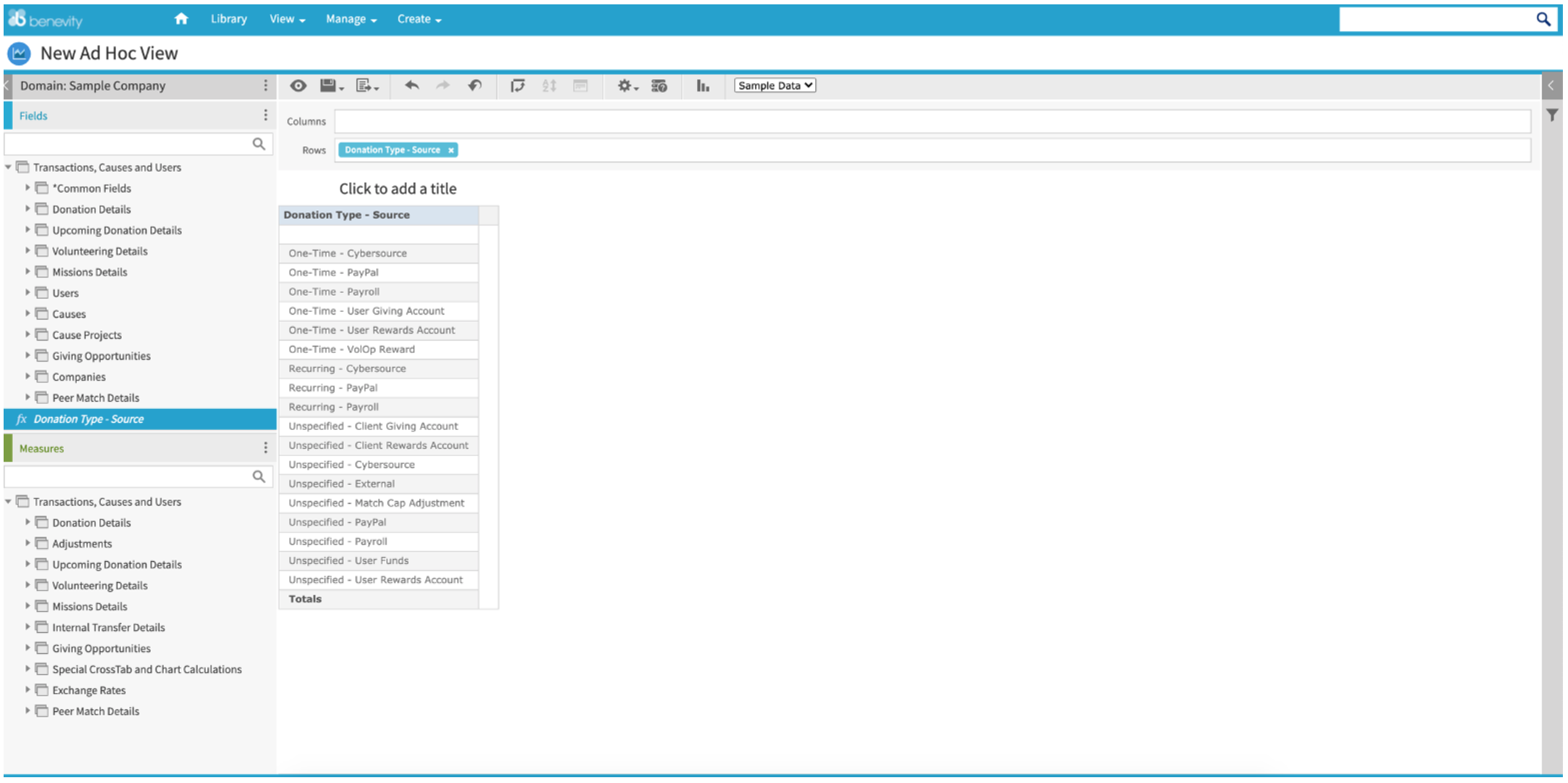The output of your new calculated field will be displayed in the crosstab.

Your new calculated field can also be used as a filter.

8. Right-click the new calculated field name in the list of Fields and select Create Filter.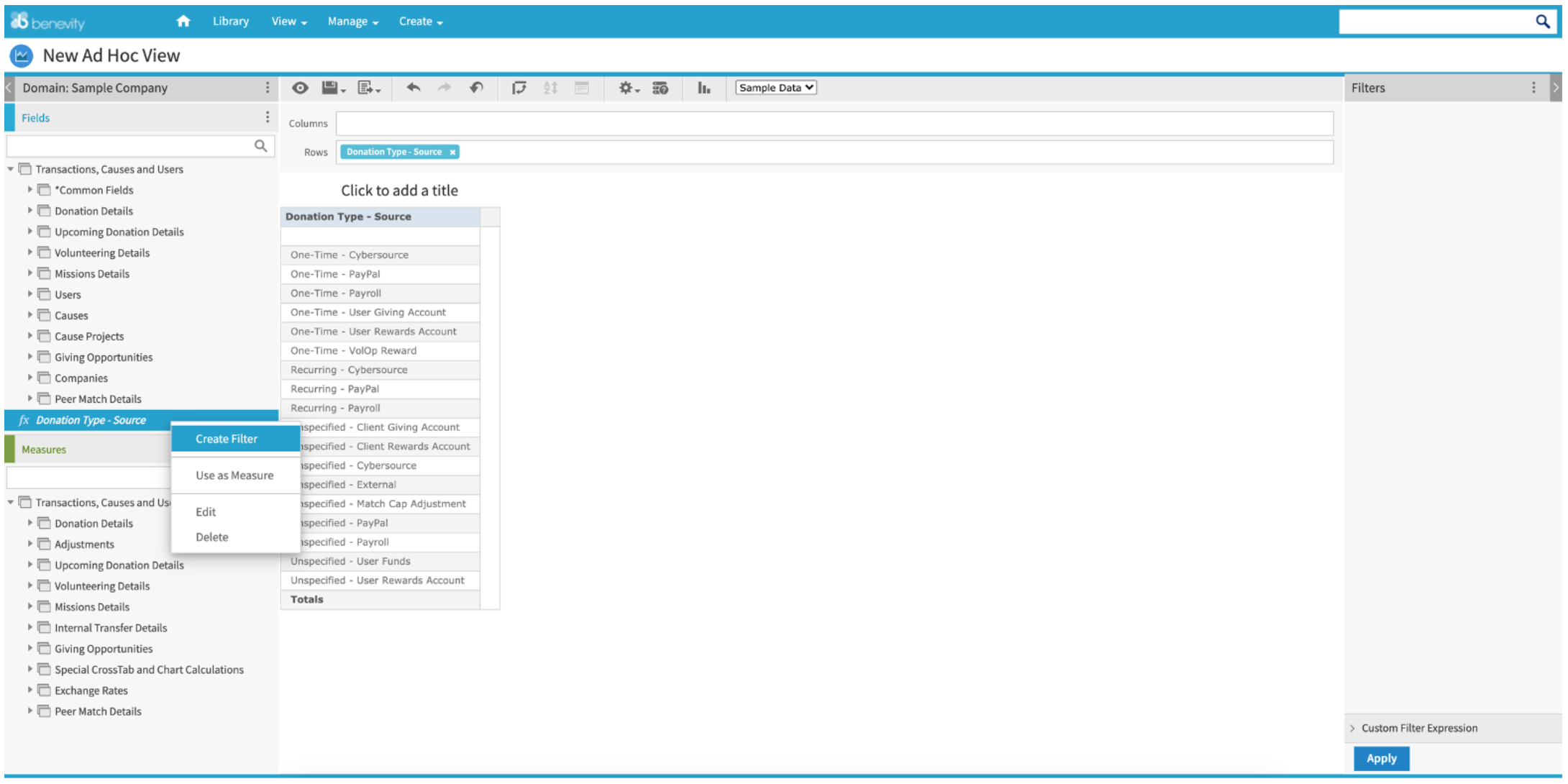You can now use your new calculated field to filter the results in your ad hoc view.

9. Select the items from the filter list you would like to include (or exclude) in the ad hoc view.

10. Click Apply.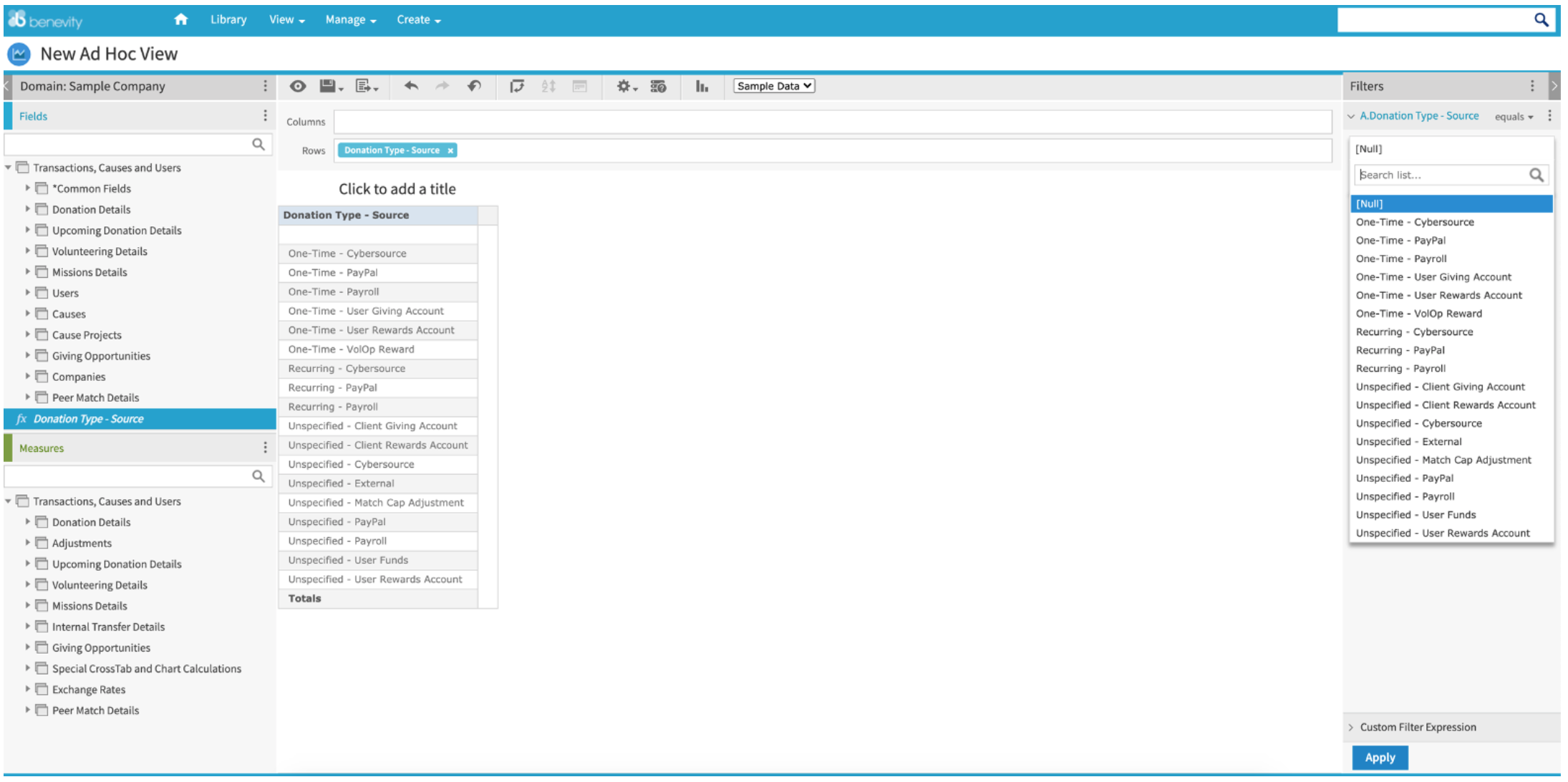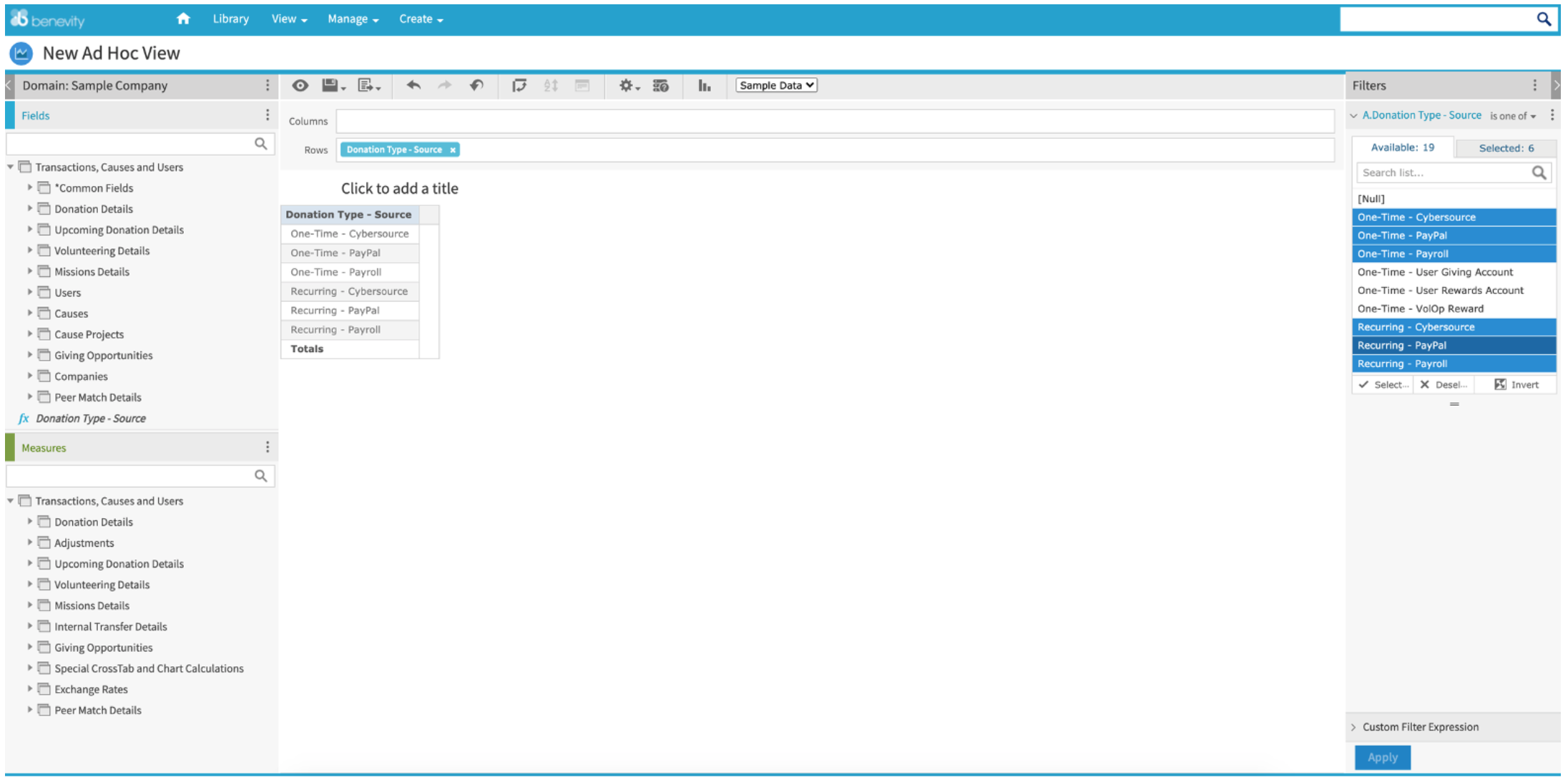### Creating a calculated measure

1. Create a new ad hoc view or open an existing ad hoc view that you would like to apply the calculated measure to.

2. In the ad hoc editor, click on the three vertical dots to the right of Measures in the left-hand pane.

3. Click Create Calculated Measure…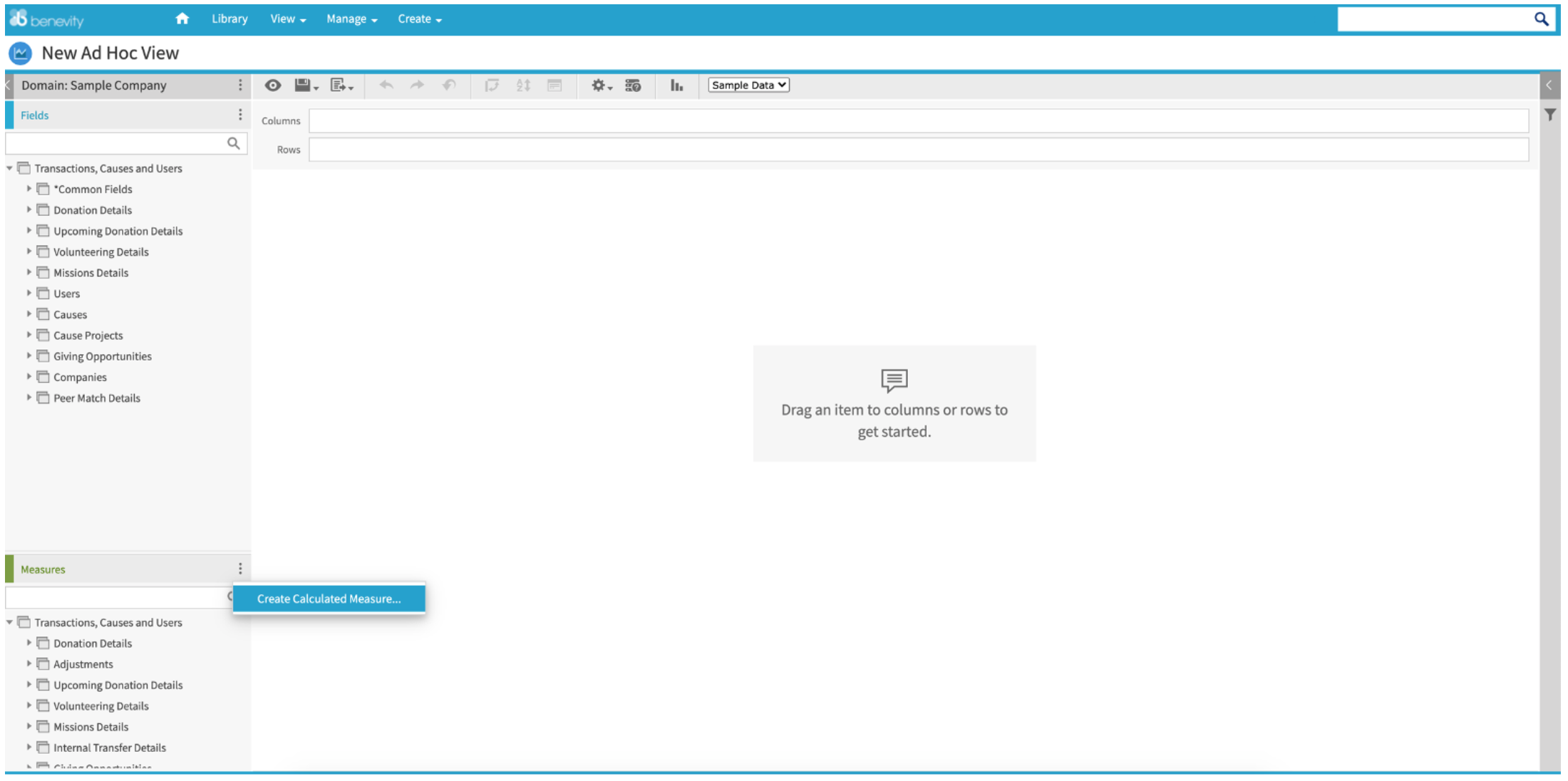In the middle of the screen, you will see the New Calculated Measure window.

In the Formula Builder tab, you can create a custom formula by selecting a field from the list of Fields and Measures below and applying a formula to the field. A list of the various Functions available is also provided. You can see a short description of your chosen function in the Function Description view to the right of the Functions list.

As well, you can include various operators in the formula:

• Arithmetic operators (+ add, - subtract, * multiply, / divide)
• Boolean operators (AND, OR, NOT, IN)
• Other operators (== equals, != not equal, > greater than, < less than, >= greater than or equal to, <= less than or equal to)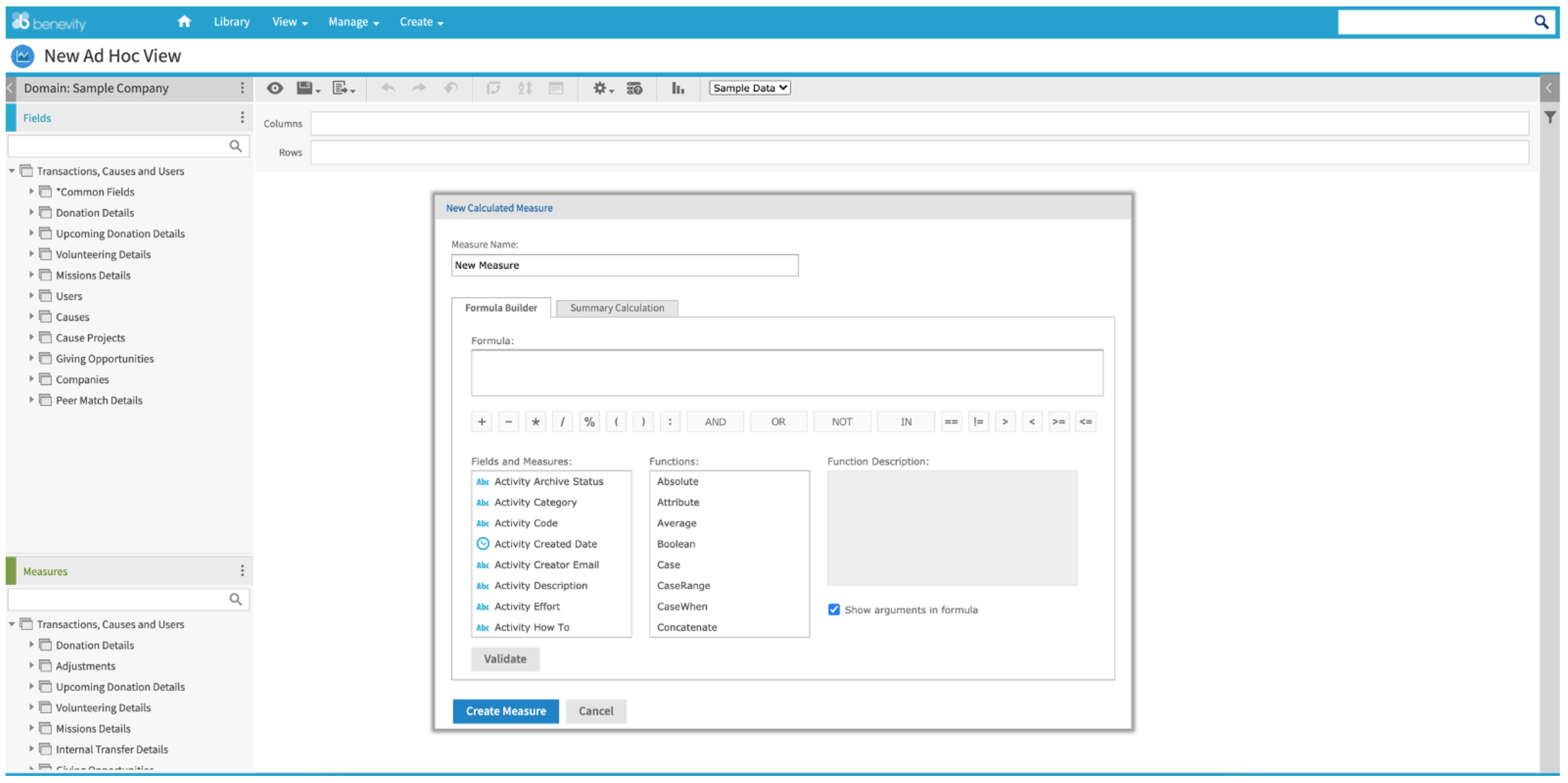5. Once you have created your calculated measure, add a Measure Name and click Validate to check that the formula has been created correctly.

6. Click Create Measure.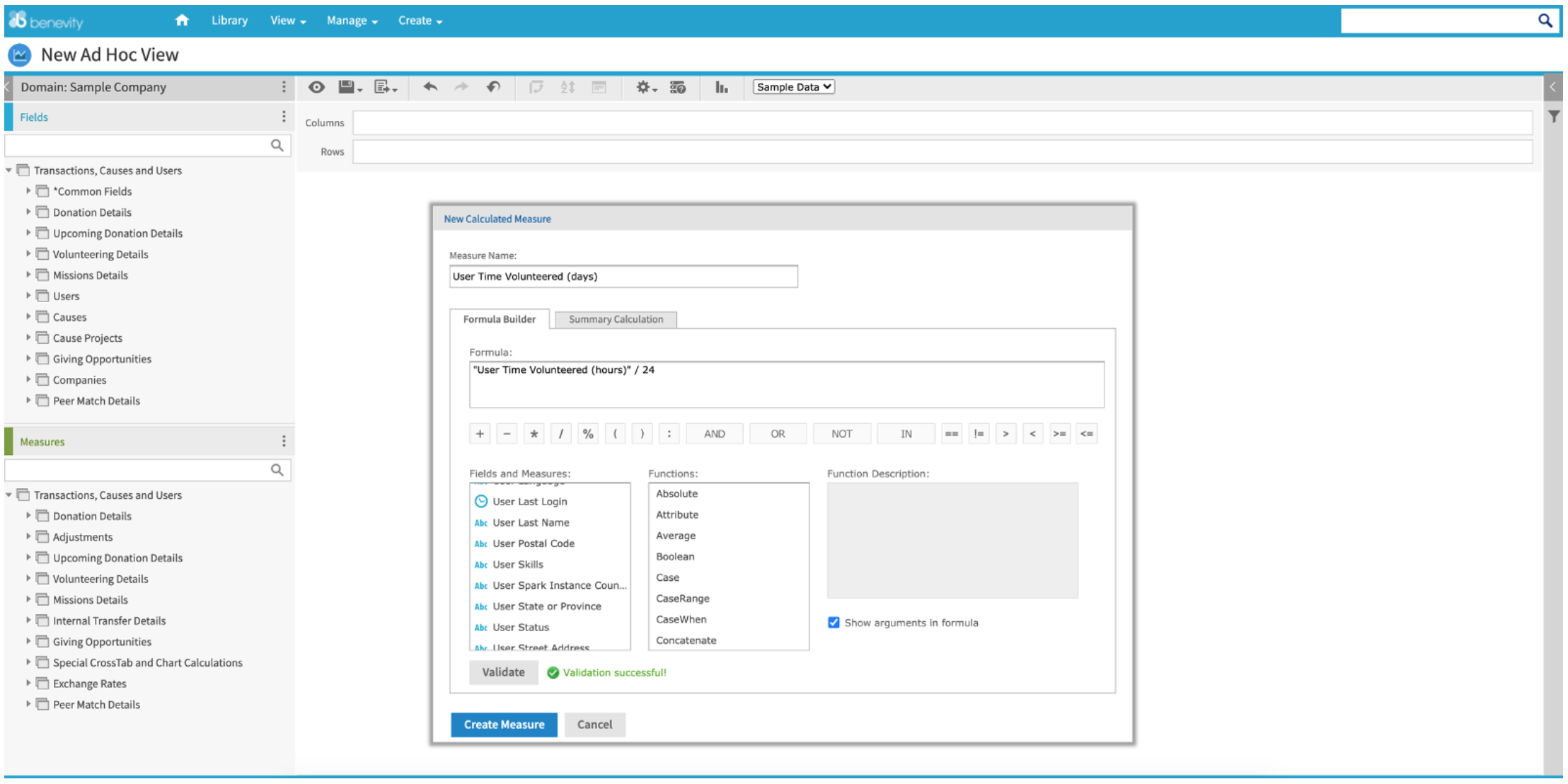Your new calculated measure will appear at the bottom of your list of Measures and can now be dragged into the Columns or Rows section of the crosstab view to the right.

7. Add the new calculated measure to the Columns or Rows in the crosstab view to the right to see the output of your new measure.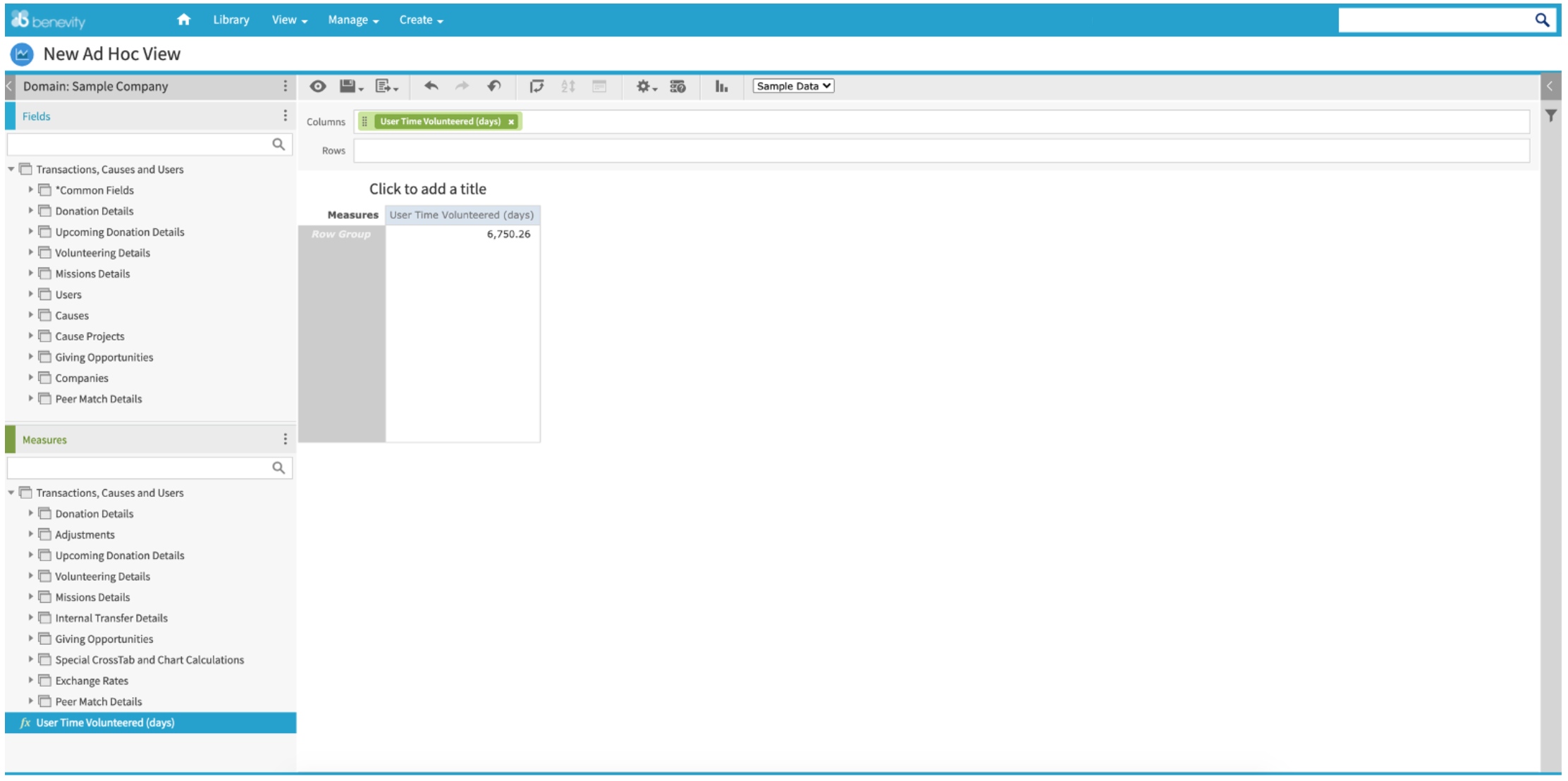The output of your new calculated measure will be displayed in the crosstab.